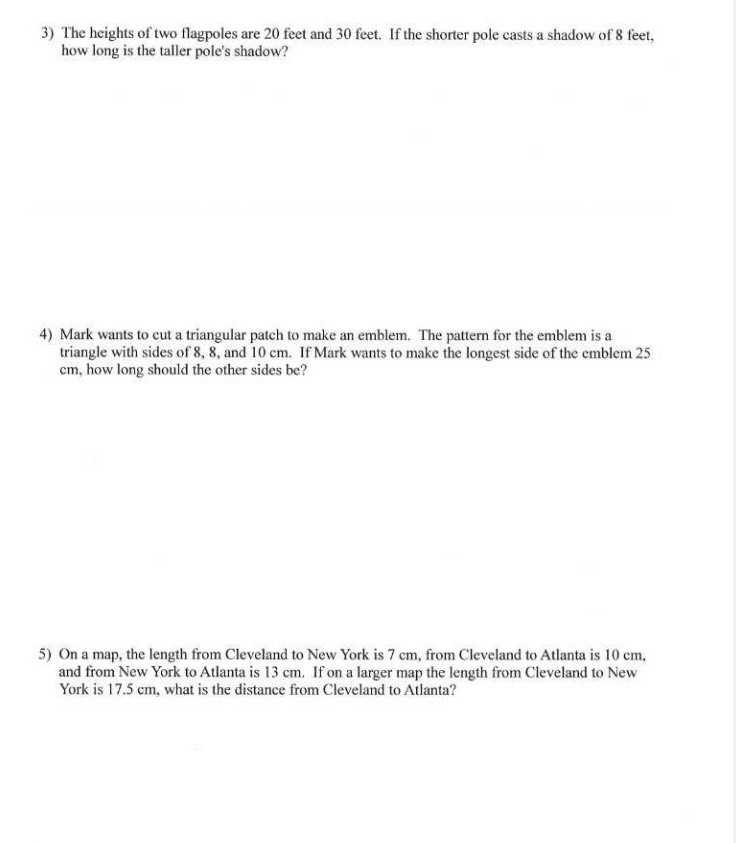### ¿Todavía tienes preguntas de matemáticas?

Pregunte a nuestros tutores expertos
Algebra
Pregunta3) The heights of two flagpoles are $$20$$ feet and $$30$$ feet. If the shorter pole casts a shadow of $$8$$ feet, how long is the taller pole's shadow?

4) Mark wants to cut a triangular patch to make an emblem. The pattern for the emblem is a triangle with sides of $$8,8$$ , and $$10 cm$$ . If Mark wants to make the longest side of the cmblem $$25$$ $$cm$$ , how long should the other sides be?

5) On a map, the length from Cleveland to New York is $$7 cm$$ , from Cleveland to Atlanta is $$10 cm$$ , and from New York to Atlanta is $$13 cm$$ . If on a larger map the length from Cleveland to New York is $$17.5 cm$$ , what is the distance from Cleveland to Atlanta?